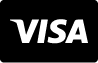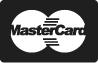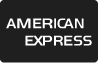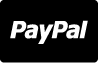Central De Ajuda
Fotos
Vetores
Vídeos
Áudios
Veja nossos planos e preços
Central De Ajuda

# Imagens - Simple problems of multiplying with oranges, mathematical problems for children who study and want to learn math and calculus; learn to multiply with fruits and numbers, oranges and figures and numerical symbols

Simple problems of multiplying with oranges, mathematical problems for children who study and want to learn math and calculus; learn to multiply with fruits and numbers, oranges and figures and numerical symbols
ID da imagem : 138297493
Tipo de Arquivo :
Direitos autorais :
9301 x 3829 px | 31 " x 12.76 " | 300dpi | JPG
Palavras-chave do Banco de Imagens
Mostrar mais
Show Less
Precisa de ajuda? Entre em contato com nosso atendimento
1 866 655 3733